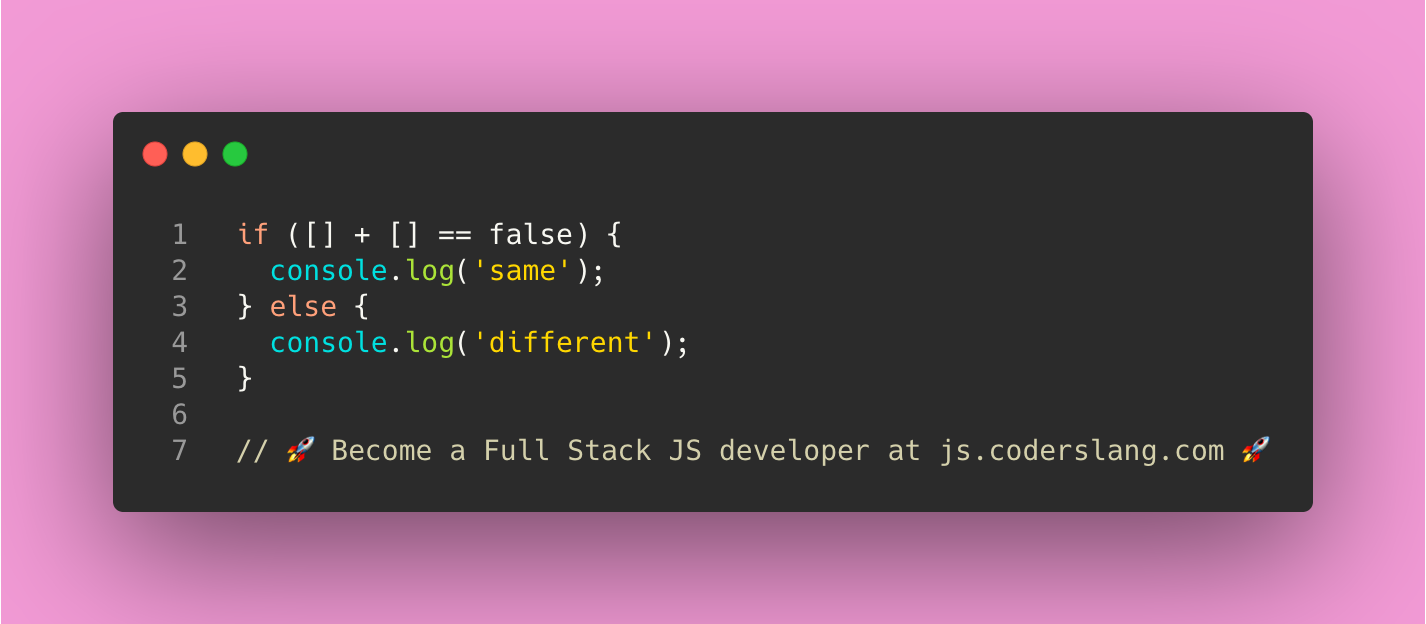Is the sum of two arrays equal to `false`?

.

.

.

.

.

.

.

.

.

.

.

.

.

.

.

.

To analyze this code snippet we need to understand how type conversion works in JS.

When we try to sum two arrays using the `+` operator, the arrays are first converted to strings and then these strings are concatenated.

An empty array `[]` is evaluated as an empty string. The sum of two empty strings is still an empty string.

Now, is an empty string equal to `false` or not?

The comparison here is done using the `==` operator. This operator is used to check `loose equality` and does implicit type conversion.

In this case, empty string and `false` are considered equal and the condition of the `if` statement will be evaluated to `true`.

If you want to use a strict comparison which respects the types of values you compare, you should use the strict equality operator `===`.

Here, you can find more information on basic math operations is JavaScript.

ANSWER: the string `same` will be logged to the console.